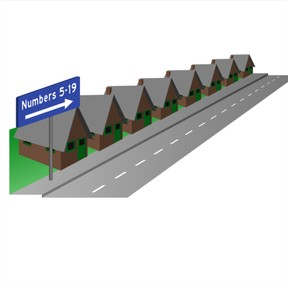Know even and odd numbers to 30

# Know even and odd numbers to 30

Know even and odd numbers to 30

No account needed.8,000 schools use Gynzy92,000 teachers use Gynzy1,600,000 students use Gynzy

## General

Students are introduced to the terms even and odd. Students learn what even and odd numbers are by skip-counting in twos.

2.OA.C.3

## Relevance

Discuss with students that it is important to know what even and odd numbers are when you want to know which side of the street a house is on. Explain that streets always have even numbers on one side, and odd numbers on the other side.

## Introduction

Count up and down to 20 with students aloud. Ask students to count with their eyes closed, and also have them start counting from a random given number up to 20 and back down to the given number. Ask students to (either in their groups, or while walking through the classroom) count 2-4-6-8-10 and 1-3-5-7-9 etc. The even and odd numbers can be dealt with in this way without naming them as even and odd numbers. An idea might be to ask the students to increase their volume as they count up. Alternate with counting down. Ask students to fill in the missing numbers.

## Development

Explain to students that there are two number lines, and that depending on which number line they are in, will say if the number is even or odd. Skip-count in twos. If you start at 0, you will always get an even number, and if you start with 1 you will always get an odd number. Count the even and odd number lines aloud together with the students to 20 , and ask them to continue counting to 30.

Check that students understand what even and odd numbers are by asking the following questions:
Which of these numbers are even? odd? How do you know?
Can you explain why 12 is an even number? And why 17 is an odd number?

## Guided Practice

Students practice with dragging even numbers to the correct column. Next they must fill in house numbers and finally a row of even and odd numbers.

## Closing

Check that students understand what even and odd numbers are and ask them to explain where they might come across them. Finish with a class game. Name a random number from 1-30. The students clap when the number is even, and remain still if it is odd.

## Teaching Tip

If students are not comfortable skip-counting in 2's, ask them to write the numbers to 30 in alternating colors, and use this as a support for learning.

### The online teaching platform for interactive whiteboards and displays in schools

• Save time building lessons

• Manage the classroom more efficiently

• Increase student engagement